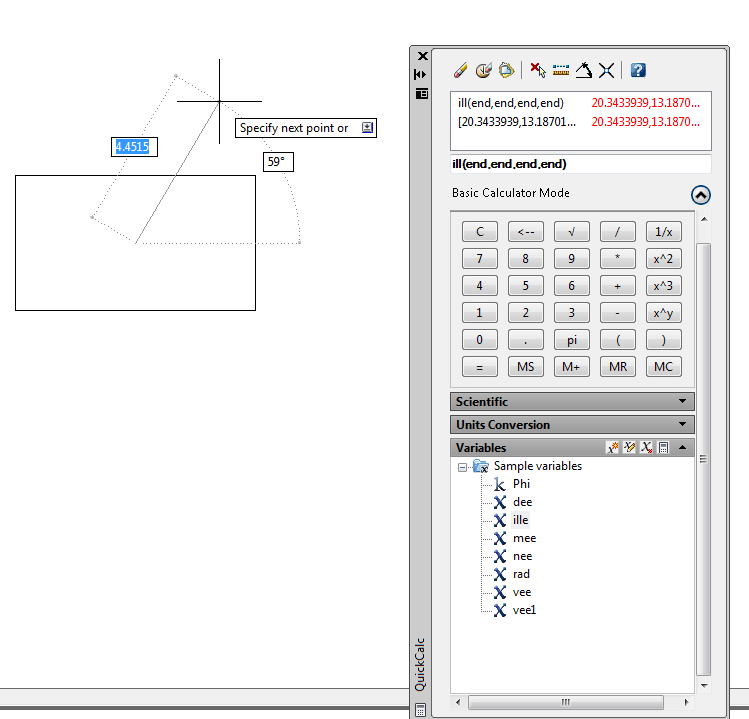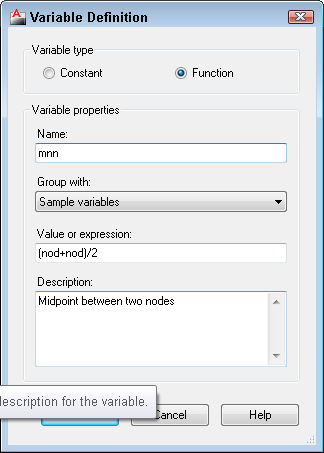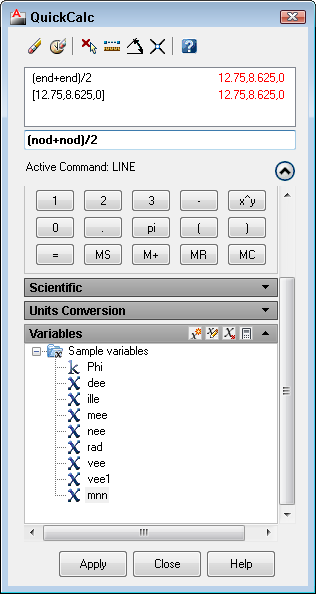The most comprehensive AutoCAD book around!# Create a custom function in AutoCAD’s calculator

AutoCAD calculator (the QUICKCALC command )is a great feature for both simple and complex calculations. One of the great features is the ability to calculate coordinates.

QuickCalc has variables (or functions) that are shortcuts to some of the more complex coordinate calculations. To use these functions, follow these steps:

1. Start a command.
2. At the prompt where you need the function, type ‘quickcalc (or ‘qc). The QuickCalc window opens.
3. From the Variables list, double-click the function that you want to place it in the Input box of the QuickCalc window.
5. Pick the required points, most commonly by object snaps. The QuickCalc window returns, and you see an absolute coordinate in the Input box.
6. Click the Apply button.
7. Continue the command.

For example, let’s say I have a rectangle and I want to start a line at its center, defined as the intersection of the two diagonal lines drawn from opposite corners. Here’s one way to do that:

1. Start the LINE command.
2. At the Specify first point: prompt, type ‘qc.
3. From the Variables list (you might have to expand the QuickCalc window to see it; then expand the Variables category), double-click ille.
5. Pick the first set of diagonal points, such as the upper-left, then the lower-right corners of the rectangle.
6. Pick the second set of diagonal points, such as the upper-right, then the lower-left corners of the rectangle.
7. Click the Apply button in the QuickCalc window.
8. Your line’s start point is now set at the center of the rectangle and you can continue the command.### Create a custom function

When you click a function, you see a tooltip giving you the full format of the function.  You can use this format to create your own functions. For example, mee (midpoint between two endpoints) is (end+end)/2. So, to find the midpoint between two nodes (rather than two endpoints), you would use (nod+nod)/2.

To save your custom function, click the New Variable button at the top of the Variables section. The Variable Definition dialog box opens, where you can define and save the variable.Then, your custom variable appears on the list and you can use it like one of the variables that come with AutoCAD. You can see mnn at the very bottom of the list.How cool is that? Have you created a custom variable? Please share it in the comments.

### Related tips

Find the midpoint between two points, the center of a triangle, and other unusual points

•Bob Lawrence
•admin